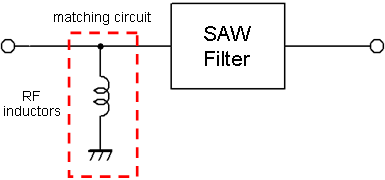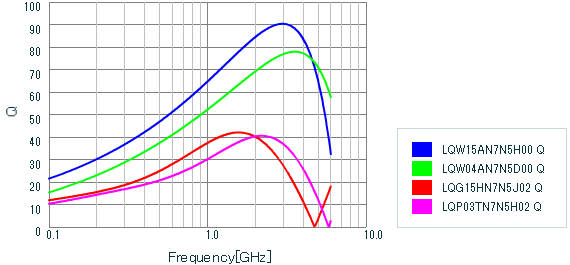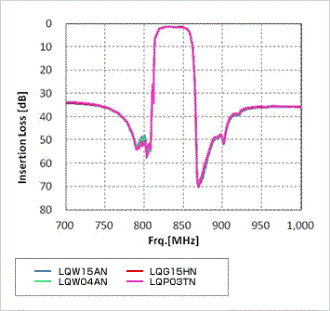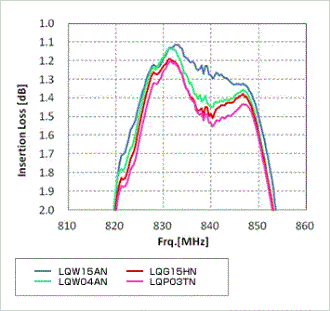# RF InductorRF Inductors - Matching

Introduction about the impedance matching of the RF inductors.
* Murata refers to inductors suitable for use in high frequency circuits as "high frequency inductors" and "RF inductors".

## 1Impedance Matching

In high frequency circuits, it is important to perform impedance matching between circuits.
Impedance matching is to match the output impedance of the sending side circuit, and the input impedance of the receiving side circuit in the signal transmission path.
By matching the impedance, the power on the sending side can be transmitted at the maximum to the receiving side.

Although a capacitor and inductor are used in the matching circuit, the actual capacitor and inductor are not ideal components, and a loss will be generated.
There is a Q to express this loss.
When the Q is higher, it shows that the capacitor and inductor have less loss.

## 2Inductor Q and High Frequency Circuit Loss

The loss in high frequency circuits is affected by the level of inductor Q used for matching circuits.
In order to confirm this, Murata's SAW filter (800 MHz pass band) and RF inductor were used to replace the RF inductor with a different Q in the matching circuit, and the insertion loss of the SAW filter was measured and compared.

In the case of this circuit, only 1 RF inductor is used in the matching circuit.Figure 1: shows the circuit diagram.

Figure 2 shows the frequency characteristics of the replaced RF inductor Q, and Table 1 shows the structure, size and Q (Typ. value at 800 MHz) .Figure 2: Comparison of RF Inductor Q (both 7.5 nH)

Table 1 Comparison of RF Inductor
Part number Structure Size (mm) Q
LQW15AN7N5H00 Wire wound 1.0×0.5 59
LQW04AN7N5D00 Wire wound 0.8×0.4 48
LQG15HN7N5J02 Multilayer 1.0×0.5 33
LQP03TN7N5H02 Film 0.6×0.3 27

* Design support tool Simsurfingprovided by Murata was used to display the graph in Figure 2.

Figure 3 shows the overall characteristics of the SAW filter when the RF inductor is replaced in the matching circuit, and Figure 4 shows the pass band characteristics.

•Figure 3: Overall Characteristics of SAW Filter
•Figure 4: Pass Band Characteristics of SAW Filter

When looking at the pass band characteristics of Figure 4, it can be confirmed that the insertion loss of the SAW filter differs by the RF inductor used.
This different level of loss is important for high frequency circuits.
From the results of this test, it is clear that when the level of the RF inductor Q is higher (low loss) the insertion loss of the SAW filter becomes less.
Accordingly, the level of the inductor loss is the level of the SAW filter loss including the matching circuit.

Keep in mind that the loss will change according to the high frequency circuit being used (SAW filter in this test), matching circuit, frequency band and etc.

## 3Inductor Tolerance and Effect on Matching Circuit

The actual inductance value of the inductor is not a continued value like 1.0 nH, 1.1 nH and 1.2nH.
When performing matching, there are cases where fine constant steps are required to make fine adjustments.
Since the tolerance of the inductance value becomes the variation in the matching, an inductor with less tolerance may be required to satisfy the necessity.
In Murata's inductors, the film type LQP series is ideal for fine constant steps, and less tolerance.

According to the above, compare and examine the Q characteristics, tolerance, size, cost and etc. to select an RF inductor for matching circuits.